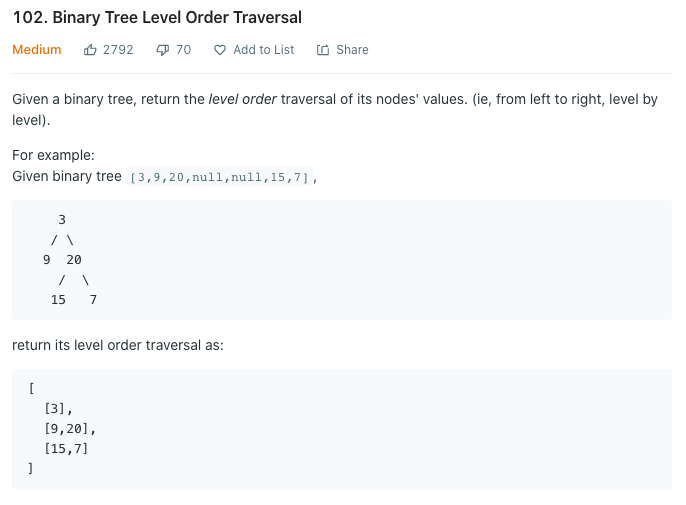# Leetcode 102. Binary Tree Level Order Traversal

0

## 알고리즘 문제풀이(Leetcode)

목록 보기
38/42# Definition for a binary tree node.
# class TreeNode:
#     def __init__(self, val=0, left=None, right=None):
#         self.val = val
#         self.left = left
#         self.right = right
class Solution:
def levelOrder(self, root: TreeNode) -> List[List[int]]:
if not root: return

q = collections.deque([root, None]) # here, None will let you know the level
tmp = []
res = []
while q:
node = q.popleft()
if node is None:
res.append(tmp)
tmp = []
if q: q.append(None)

else:
tmp.append(node.val)
if node.left: q.append(node.left)
if node.right: q.append(node.right)

return res

https://leetcode.com/problems/binary-tree-level-order-traversal/